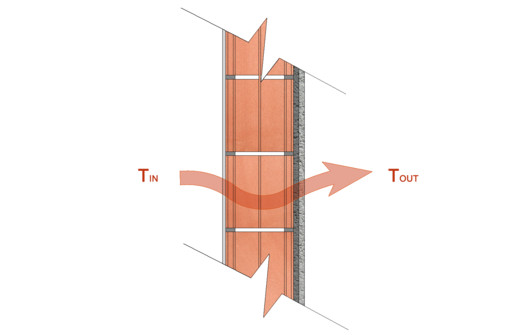# How to Calculate the Thermal Transmittance (U-Value) in the Envelope of a Building

How to Calculate the Thermal Transmittance (U-Value) in the Envelope of a Building

When designing the envelope of projects, we must pay special attention to each of the elements that comprise it, since each of these layers has specific qualities that will be decisive in the thermal behavior of our building as a whole.

If we divide 1 m2 of our envelope by the temperature difference between its faces, we will obtain a value that corresponds to the thermal transmittance, also called U-Value. This value tells us a building's level of thermal insulation in relation to the percentage of energy that passes through it; if the resulting number is low we will have a well-isolated surface and, on the contrary, a high number alerts us of a thermally deficient surface.

Expressed in W/m²·K, the U-Value depends on the thermal resistance of each of the elements that make up the surface (the percentage in which a building element is opposed to the passage of heat), and this, in particular, obeys the thickness of each layer and its thermal conductivity (ability to conduct the heat of each material). Let's review the formulas necessary to calculate the thermal transmittance of our envelope.

### Thermal Envelope

The thermal envelope is defined as the "skin" of the building, which protects the thermal and acoustic comfort of its interiors. This is made up of its opaque walls (walls, floors, ceilings), its operable elements (doors and windows), and its thermal bridges, which are all those points that allow heat to pass more easily (points with geometric variations or changes of materials).

In the case of envelopes that are not completely homogeneous in their extension, for example in metal or wooden structures, it's possible to perform differentiated calculations for different areas and obtain a greater accuracy in the results. The total is then calculated on the approximate percentages to each of them, which can be found in the local standards and regulations corresponding to the location of the project.

## Thermal Transmittance Calculation

The general formula for calculating the U-Value is:

## U = 1/Rt

Where:

• U = Thermal Transmittance (W/m²·K)*
• Rt = Total Thermal Resistance of the element composed of layers (m²·K/W), obtained according to:

## Rt = Rsi + R1 + R2 + R3 + ... + Rn + Rse

Where:

• Rsi = Interior Surface Thermal Resistance (according to the norm by climatic zone)
• Rse = Exterior Surface Thermal Resistance (according to the norm by climatic zone)
• R1, R2, R3, Rn = Thermal Resistance of each layer, which is obtained according to:

## R = D / λ

Where:

The Thermal Transmittance is inversely proportional to the Thermal Resistance: the greater the resistance of the materials that make up an envelope, the lower the amount of heat that is lost through it.

## R = 1/U

### Climatic Zones

When obtaining our U-Value, we must compare it with the value of the maximum (or limit) thermal transmittance specified for the climatic zone in which our project is located, in winter and summer. This number has been determined by official local regulations, which you must review carefully to ensure proper functioning.

* W = Power (Watts) - K = Temperature difference (Kelvin)## #Tags

Cite: Franco, José Tomás. "How to Calculate the Thermal Transmittance (U-Value) in the Envelope of a Building" [¿Cómo calcular la transmitancia térmica (Valor U) en la envolvente material de un edificio?] 29 Jul 2018. ArchDaily. Accessed . <https://www.archdaily.com/898843/how-to-calculate-the-thermal-transmittance-u-value-in-the-envelope-of-a-building> ISSN 0719-8884

## Top #Tags